## RS Aggarwal Class 7 Solutions Chapter 2 Fractions Ex 2A

These Solutions are part of RS Aggarwal Solutions Class 7. Here we have given RS Aggarwal Solutions Class 7 Chapter 2 Fractions Ex 2A.

Other Exercises

Question 1.
Solution: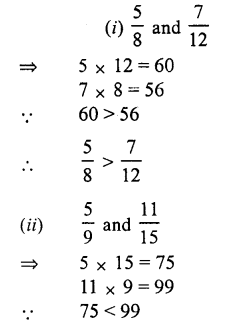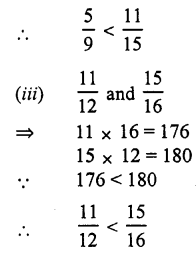Question 2.
Solution: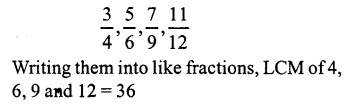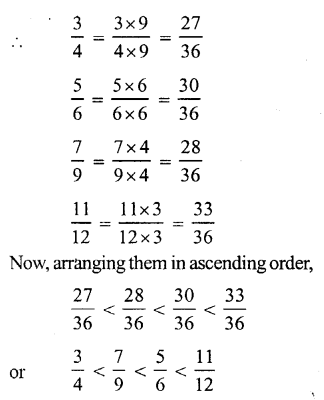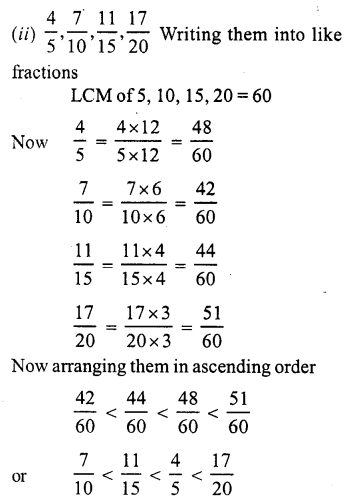Question 3.
Solution: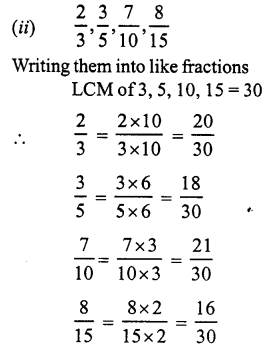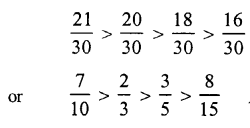Question 4.
Solution:
Reenu get $$\frac { 2 }{ 7 }$$ of an apple while Sonal gets $$\frac { 4 }{ 5 }$$ part of it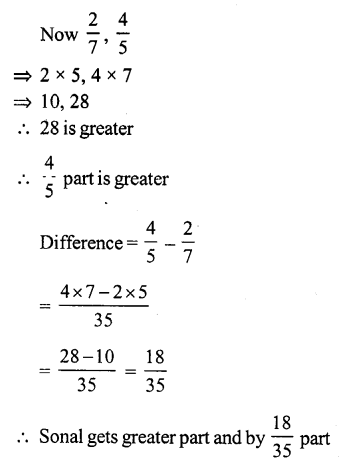Question 5.
Solution: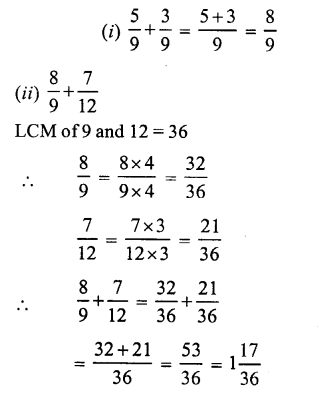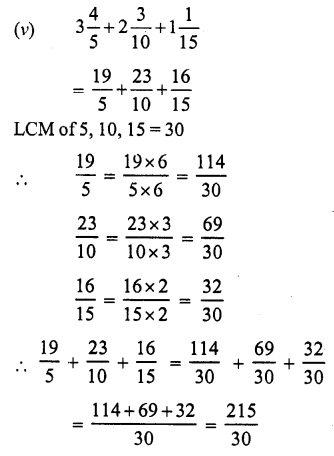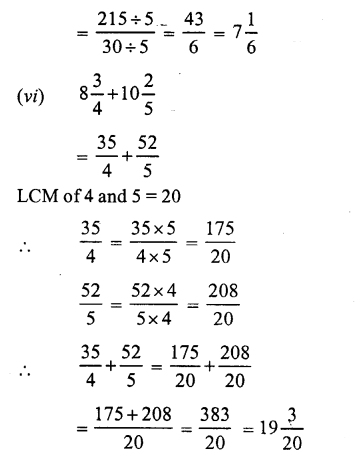Question 6.
Solution: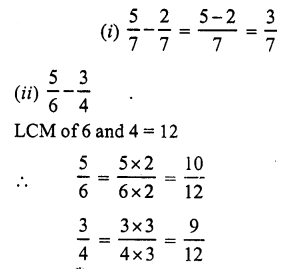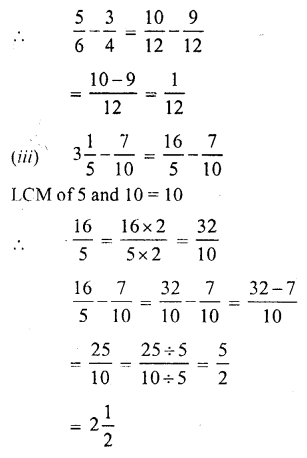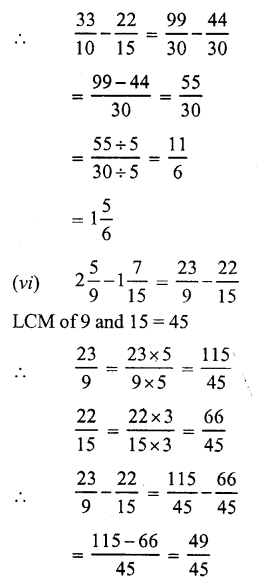Question 7.
Solution: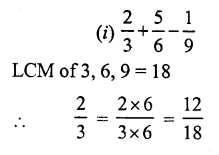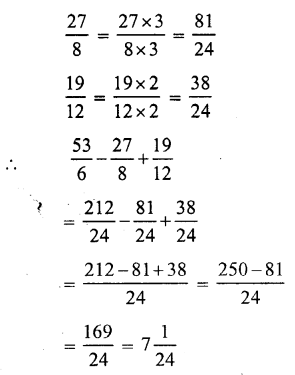Question 8.
Solution: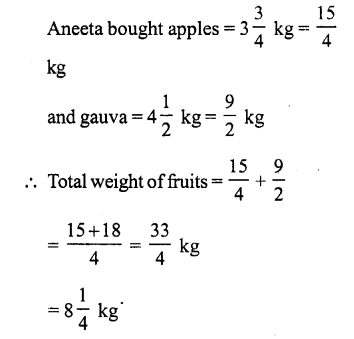Question 9.
Solution: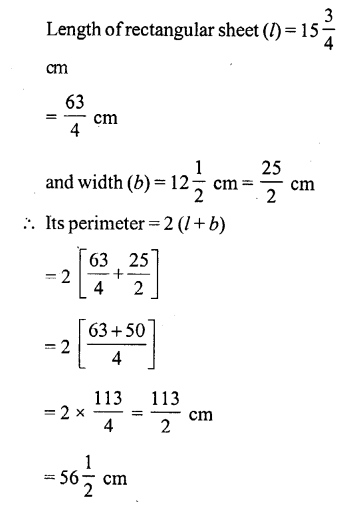Question 10.
Solution:Question 11.
Solution:
For finding the required fraction, we have to subtract 7$$\frac { 3 }{ 5 }$$ from 18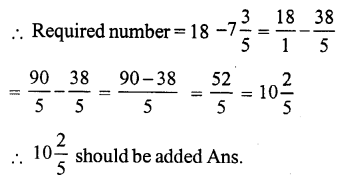Question 12.
Solution:
For finding the required fraction we should subtract 7$$\frac { 4 }{ 15 }$$ from 8$$\frac { 2 }{ 5 }$$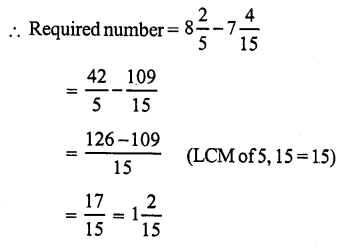Question 13.
Solution: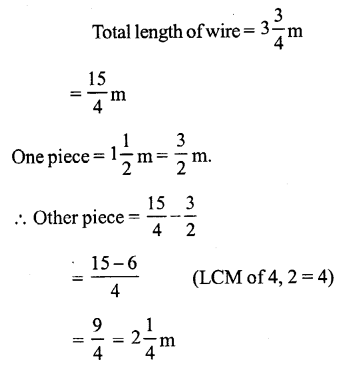Question 14.
Solution: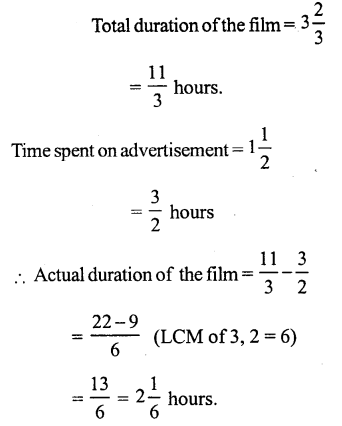Question 15.
Solution: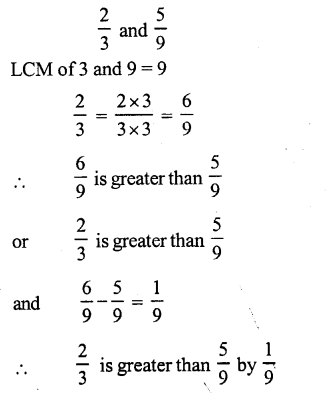Question 16.
Solution: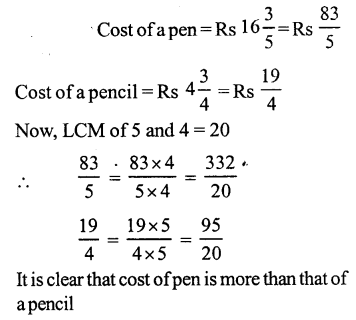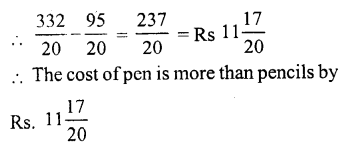Hope given RS Aggarwal Solutions Class 7 Chapter 2 Fractions Ex 2A are helpful to complete your math homework.

If you have any doubts, please comment below. Learn Insta try to provide online math tutoring for you.